﻿ 高温中压蒸汽系统球阀热应力仿真计算及寿命评估 Simulation and Life Evaluation of Thermal Stress of Ball Valve in High Temperature Medium Pressure Steam System

Applied Physics
Vol.08 No.06(2018), Article ID:25473,7 pages
10.12677/APP.2018.86037

Simulation and Life Evaluation of Thermal Stress of Ball Valve in High Temperature Medium Pressure Steam System

Zhulin Dong1, Jianjun Liu2, Shengjian Yu3

1Equipment Procurement Center of Chinese Navy Equipment Department, Beijing

2China Ship Development and Design Center, Wuhan Hubei

3Dalian Shipbuilding Industry Co. Ltd., Dalian Liaoning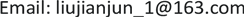Received: Jun. 3rd, 2018; accepted: Jun. 20th, 2018; published: Jun. 27th, 2018ABSTRACT

Based on the calculation results of the steady-state temperature field, a transient temperature calculation is performed, and then the thermal stress of the entire valve system is calculated based on the results of the transient temperature field. For the valve body local concentrated stress up to 278 MPa, the thermal stress of other components are less than the allowable stress of the material. The fatigue resistance of the valve shell was analyzed.

Keywords:Ball Valve, High Temperature, Heat Stress, Life Assessment

1海军装备部装备采购中心，北京

2中国舰船研究设计中心，湖北 武汉

3大连船舶重工集团有限公司，辽宁 大连1. 引言

2. 热应力数学模型

1) 轴对称运动微分方程

$\left\{\begin{array}{l}\frac{\partial {\sigma }_{x}}{{\partial }_{x}}+\frac{\partial {\tau }_{rx}}{{\partial }_{x}}+\frac{{\tau }_{rx}}{r}+X=\rho \frac{{\partial }^{2}u}{\partial {t}^{2}}\\ \frac{\partial {\sigma }_{r}}{{\partial }_{r}}+\frac{\partial {\tau }_{xr}}{{\partial }_{x}}+\frac{{\sigma }_{r}-{\sigma }_{\theta }}{r}+R=\rho \frac{{\partial }^{2}\upsilon }{\partial {t}^{2}}\end{array}$ (1)

2) 几何方程

$\left\{\epsilon \right\}={\left[{\epsilon }_{x},{\epsilon }_{r},{\epsilon }_{\theta },{\epsilon }_{xr}\right]}^{\text{T}}={\left[\frac{\partial u}{\partial x},\frac{\partial \upsilon }{\partial r},\frac{\upsilon }{r},\frac{\partial \upsilon }{\partial x}+\frac{\partial u}{\partial r}\right]}^{\text{T}}$ (2)

3) 物理方程

$\left\{\epsilon \right\}={\left[{\epsilon }_{x0},{\epsilon }_{r0},{\epsilon }_{\theta 0},{\epsilon }_{xr0}\right]}^{\text{T}}={\left[\alpha \Delta T,\alpha \Delta T,\alpha \Delta T,0\right]}^{\text{T}}$ (3)

$\left\{\begin{array}{l}{\sigma }_{x}=\frac{E}{\left(1+\mu \right)\left(1-2\mu \right)}\left[\left(1+\mu \right)\left({\epsilon }_{x}-{\epsilon }_{x0}\right)+\mu \left({\epsilon }_{r}-{\epsilon }_{r0}\right)+\mu \left({\epsilon }_{\theta }-{\epsilon }_{\theta 0}\right)\right]\\ {\sigma }_{r}=\frac{E}{\left(1+\mu \right)\left(1-2\mu \right)}\left[\mu \left({\epsilon }_{x}-{\epsilon }_{x0}\right)+\left(1-\mu \right)\left({\epsilon }_{r}-{\epsilon }_{r0}\right)+\mu \left({\epsilon }_{\theta }-{\epsilon }_{\theta 0}\right)\right]\\ {\sigma }_{\theta }=\frac{E}{\left(1+\mu \right)\left(1-2\mu \right)}\left[\mu \left({\epsilon }_{x}-{\epsilon }_{x0}\right)+\mu \left({\epsilon }_{r}-{\epsilon }_{r0}\right)+\left(1-\mu \right)\left({\epsilon }_{\theta }-{\epsilon }_{\theta 0}\right)\right]\\ {\tau }_{xy}=\frac{E}{2\left(1+\mu \right)}{\nu }_{xy}\end{array}$ (4)

3. 几何模型

4. 热应力计算及分析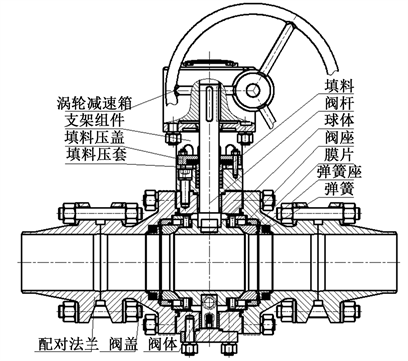Figure 1. Structure of ball valve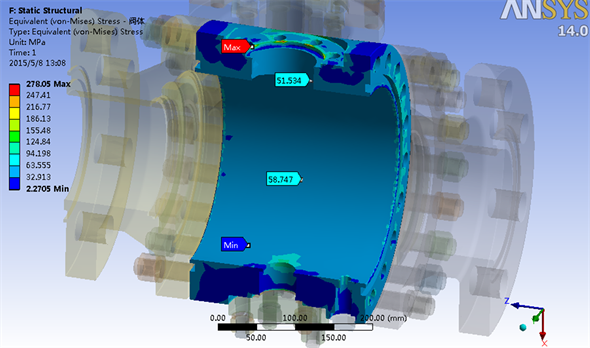Figure 2. Body thermal stress distribution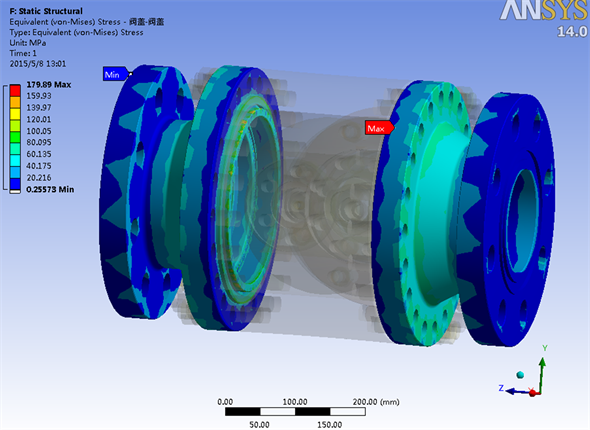Figure 3. Bonnet-Valve Cover Thermal Stress Distribution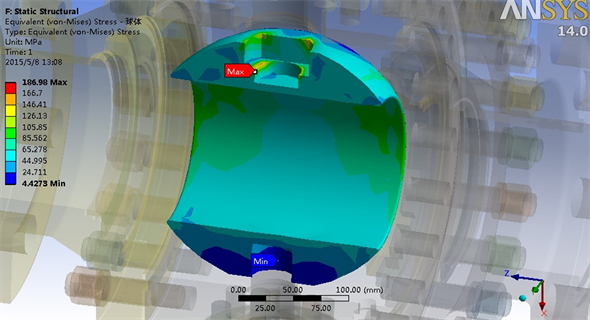Figure 4. Ball thermal stress distributionFigure 5. Valve stem thermal stress distribution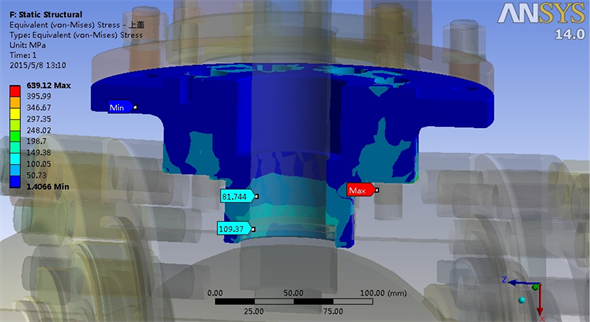Figure 6. Top cover thermal stress distribution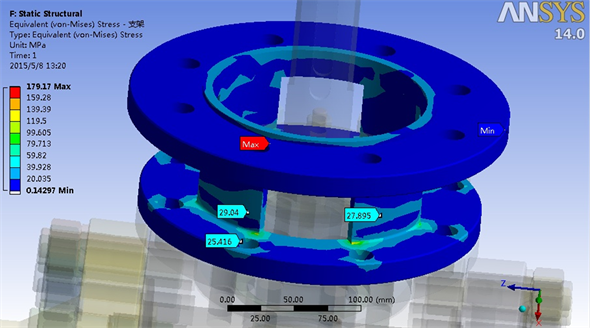Figure 7. Bracket thermal stress distribution

5. 疲劳分析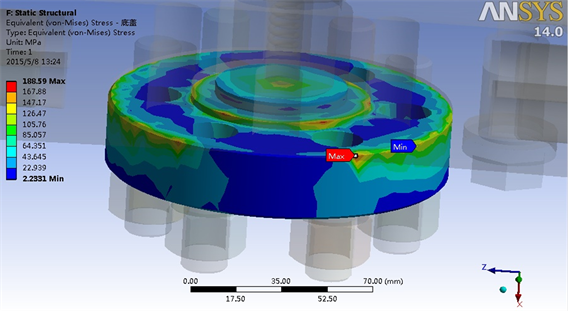Figure 8. Bottom cover thermal stress distribution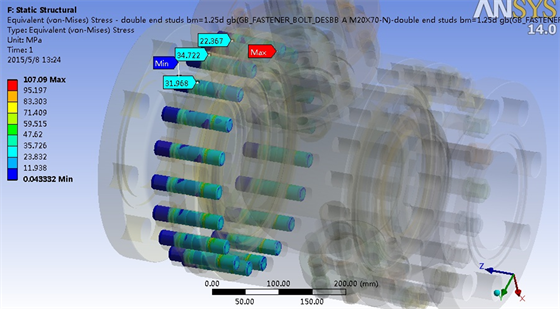Figure 9. Body-bonnet connection bolt thermal stress distribution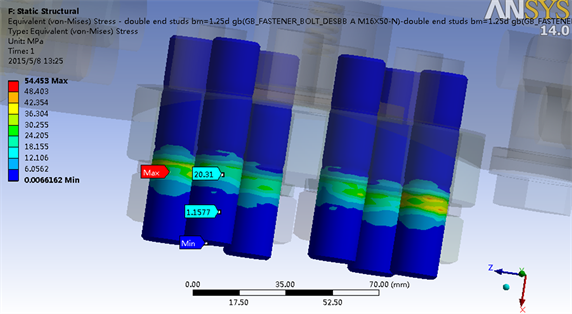Figure 10. Body-bottom cover connecting bolt thermal stress distribution

6. 结论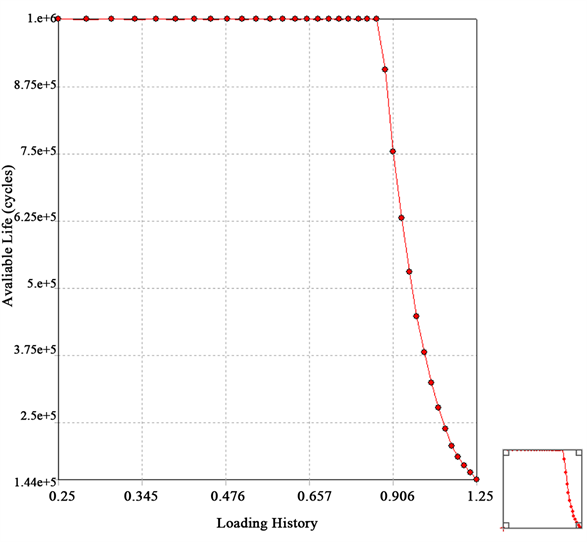Figure 11. Fatigue sensitivity curve

1) 阀门能够承受高温载荷，计算得到的主要承压件均低于材料的许用应力，满足强度要求。

2) 该阀门可在设计工况下启闭144,000次，保证了阀门承压边界的完整性。

Simulation and Life Evaluation of Thermal Stress of Ball Valve in High Temperature Medium Pressure Steam System[J]. 应用物理, 2018, 08(06): 282-288. https://doi.org/10.12677/APP.2018.86037

1. 1. 唐金兰, 余佳, 等. 双向旋转变喉面流量调节阀热应力分析[J]. 推进技术, 2011, 32(4): 591-596.

2. 2. 李金海, 熊滨生, 等. 微水节能热风阀阀板的热应力分析[J]. 中原工学院学报, 2009, 20(1): 5-7.

3. 3. 周明钢, 董中杰. 超临界600MW汽轮机组阀门启动热应力数值分析[J]. 汽轮机技术, 2013, 55(6): 420-430.

4. 4. 蒋建斐, 忻建华, 等. 660MW超临界汽轮机机组进汽阀热应力优化[J]. 热力透平, 2014, 43(4): 281-286.

5. 5. 刘金梁, 刘明, 等. 阀门热应力分析与研究[J]. 阀门, 2012(2): 12-13.